# The angle of lines

Calculate the angle of two lines y=x-21 and y=-2x+14

Correct result:

α =  -108.4 °

#### Solution:

$\alpha = \dfrac{180}{\pi} \arctan( \dfrac{ -2 -1}{ 1+1\cdot (-2)}) = -108.4 ^\circ$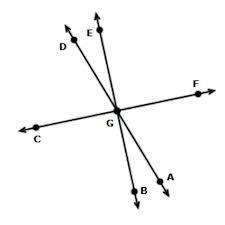Our examples were largely sent or created by pupils and students themselves. Therefore, we would be pleased if you could send us any errors you found, spelling mistakes, or rephasing the example. Thank you!

Please write to us with your comment on the math problem or ask something. Thank you for helping each other - students, teachers, parents, and problem authors.Tips to related online calculators
For Basic calculations in analytic geometry is helpful line slope calculator. From coordinates of two points in the plane it calculate slope, normal and parametric line equation(s), slope, directional angle, direction vector, the length of segment, intersections the coordinate axes etc.
Most natural application of trigonometry and trigonometric functions is a calculation of the triangles. Common and less common calculations of different types of triangles offers our triangle calculator. Word trigonometry comes from Greek and literally means triangle calculation.

## Next similar math problems:

• Angle between lines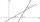Calculate the angle between these two lines: ? ?
• AngleA straight line p given by the equation ?. Calculate the size of angle in degrees between line p and y-axis.
• Find the 10Find the value of t if 2tx+5y-6=0 and 5x-4y+8=0 are perpendicular, parallel, what angle does each of the lines make with the x-axis, find the angle between the lines?
• SlopeFind the slope of the line: x=t and y=1+t.
• Supplementary angles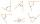One of the supplementary angles are three times larger than the other. What size is larger of supplementary angles?
• Reference angleFind the reference angle of each angle:
• Slope formFind the equation of a line given the point X(8, 1) and slope -2.8. Arrange your answer in the form y = ax + b, where a, b are the constants.
• Three points 4The line passed through three points - see table: x y -6 4 -4 3 -2 2 Write line equation in y=mx+b form
• LineLine p passing through A[-10, 6] and has direction vector v=(3, 2). Is point B[7, 30] on the line p?
• V - slopeThe slope of the line whose equation is -3x -9 = 0 is
• Perpendicular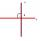Determine the slope of the line perpendicular to the line p: y = -x +4.
• Lie/do not lie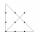The function is given by the rule f(x) = 8x+16. Determine whether point D[-1; 8] lies on this function. Solve graphically or numerically and give reasons for the your answer.
• LineIt is true that the lines that do not intersect are parallel?
• Vector - basic operationsThere are given points A [-9; -2] B [2; 16] C [16; -2] and D [12; 18] a. Determine the coordinates of the vectors u=AB v=CD s=DB b. Calculate the sum of the vectors u + v c. Calculate difference of vectors u-v d. Determine the coordinates of the vector w
• Coordinates of vector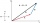Determine the coordinate of a vector u=CD if C(19;-7) and D(-16;-5)
• Profit gainIf 5% more is gained by selling an article for Rs. 350 than by selling it for Rs. 340, the cost of the article is:
• WalkingOf the 450 students at the school, 432 walks to school. What percentage is this?